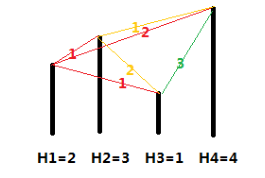# P1081 开车旅行

• 2.4K通过
• 8K提交
• 题目提供者 CCF_NOI
• 评测方式 云端评测
• 标签 倍增 NOIp提高组 2012 高性能
• 难度 省选/NOI-
• 时空限制 1000ms / 128MB
• 提示：收藏到任务计划后，可在首页查看。

## 题目描述

小$A$ 和小$B$ 决定利用假期外出旅行，他们将想去的城市从 $1$到 $N$ 编号，且编号较小的城市在编号较大的城市的西边，已知各个城市的海拔高度互不相同，记城市 $i$的海拔高度为$H_i$，城市 $i$和城市$j$之间的距离 $d_[i,j]$恰好是这两个城市海拔高度之差的绝对值，即$d_[i,j]=|H_i-H_j|$。

旅行过程中，小 $A$和小 $B$ 轮流开车，第一天小 $A$ 开车，之后每天轮换一次。他们计划选择一个城市 $S$ 作为起点，一直向东行驶，并且最多行驶$X$ 公里就结束旅行。小$A$ 和小 $B$的驾驶风格不同，小 $B$总是沿着前进方向选择一个最近的城市作为目的地，而小$A$总是沿着前进方向选择第二近的城市作为目的地（注意：本题中如果当前城市到两个城市的距离相同，则认为离海拔低的那个城市更近）。如果其中任何一人无法按照自己的原则选择目的城市，或者到达目的地会使行驶的总距离超出 $X$ 公里，他们就会结束旅行。

在启程之前，小 $A$想知道两个问题：

1. 对于一个给定的 $X=X_0$，从哪一个城市出发，小$A$ 开车行驶的路程总数与小$B$ 行驶的路程总数的比值最小（如果小 $B$ 的行驶路程为$0$，此时的比值可视为无穷大，且两个无穷大视为相等）。如果从多个城市出发，小$A$ 开车行驶的路程总数与小 $B$行驶的路程总数的比值都最小，则输出海拔最高的那个城市。
2. 对任意给定的 $X=X_i$和出发城市$S_i$，小 $A$ 开车行驶的路程总数以及小 $B$行驶的路程总数。

## 输入输出格式

输入格式：

第一行包含一个整数 $N$，表示城市的数目。

第二行有 $N$个整数，每两个整数之间用一个空格隔开，依次表示城市 $1$ 到城市$N$的海拔高度，即 $H_1,H_2,…,H_n$，且每个 $H_i$都是不同的。

第三行包含一个整数$X_0$。

第四行为一个整数 $M$，表示给定 $M$组 $S_i$和$X_i$。

接下来的 $M$ 行，每行包含 $2$ 个整数 $S_i$和 $X_i$，表示从城市$S_i$出发，最多行驶 $X_i$公里。

输出格式：

输出共$M+1$行。

第一行包含一个整数 $S_0$，表示对于给定的 $X_0$，从编号为 $S_0$ 的城市出发，小 $A$开车行驶的路程总数与小$B$ 行驶的路程总数的比值最小。

接下来的 $M$行，每行包含$2$ 个整数，之间用一个空格隔开，依次表示在给定的 $S_i$和$X_i$下小 $A$ 行驶的里程总数和小 $B$ 行驶的里程总数。

## 输入输出样例

输入样例#1： 复制
4
2 3 1 4
3
4
1 3
2 3
3 3
4 3

输出样例#1： 复制
1
1 1
2 0
0 0
0 0 
输入样例#2： 复制
10
4 5 6 1 2 3 7 8 9 10
7
10
1 7
2 7
3 7
4 7
5 7
6 7
7 7
8 7
9 7
10 7
输出样例#2： 复制
2
3 2
2 4
2 1
2 4
5 1
5 1
2 1
2 0
0 0
0 0

## 说明

【输入输出样例1说明】各个城市的海拔高度以及两个城市间的距离如上图所示。

如果从城市 $1$ 出发，可以到达的城市为 $2,3,4$，这几个城市与城市 $1$ 的距离分别为 $1,1,2$，但是由于城市 $3$ 的海拔高度低于城市 $2$，所以我们认为城市 $3$ 离城市 $1$ 最近，城市 $2$ 离城市 $1$ 第二近，所以小A会走到城市$2$。到达城市$2$后，前面可以到达的城市为$3,4$，这两个城市与城市$2$的距离分别为$2,1$，所以城市$4$离城市$2$最近，因此小B会走到城市$4$。到达城市$4$后，前面已没有可到达的城市，所以旅行结束。

如果从城市 $2$ 出发，可以到达的城市为 $3,4$，这两个城市与城市$2$的距离分别为 $2,1$，由于城市 $3$ 离城市 $2$ 第二近，所以小A会走到城市$3$。到达城市$3$后，前面尚未旅行的城市为$4$，所以城市$4$离城市$3$最近，但是如果要到达城市$4$，则总路程为 $2+3=5>3$，所以小B会直接在城市$3$结束旅行。

如果从城市 $3$ 出发，可以到达的城市为 $4$，由于没有离城市$3$第二近的城市，因此旅行还未开始就结束了。

如果从城市 $4$ 出发，没有可以到达的城市，因此旅行还未开始就结束了。

【输入输出样例2说明】

当 $X=7$ 时，如果从城市 $1$ 出发，则路线为$1 \to 2 \to 3 \to 8 \to 9$，小A走的距离为$1+2=3$，小B走的距离为$1+1=2$。（在城市$1$时，距离小A最近的城市是$2$和$6$，但是城市$2$的海拔更高，视为与城市$1$第二近的城市，所以小A最终选择城市$2$；走到$9$后，小A只有城市$10$可以走，没有第$2$选择可以选，所以没法做出选择，结束旅行）

如果从城市$2$出发，则路线为$2 \to 6 \to 7$，小A和小B走的距离分别为$2,4$。

如果从城市$3$出发，则路线为$3 \to 8 \to 9$，小A和小B走的距离分别为$2,1$。

如果从城市$4$出发，则路线为$4 \to 6 \to 7$，小A和小B走的距离分别为$2,4$。

如果从城市$5$出发，则路线为$5 \to 7 \to 8$，小A和小B走的距离分别为$5,1$。

如果从城市$6$出发，则路线为$6 \to 8 \to 9$，小A和小B走的距离分别为$5,1$。

如果从城市$7$出发，则路线为$7 \to 9 \to 10$，小A和小B走的距离分别为$2,1$。

如果从城市$8$出发，则路线为$8 \to 10$，小A和小B走的距离分别为$2,0$。

如果从城市$9$出发，则路线为$9$，小A和小B走的距离分别为$0,0$（旅行一开始就结束了）。

如果从城市$10$出发，则路线为$10$，小A和小B走的距离分别为$0,0$。

从城市$2$或者城市$4$出发小A行驶的路程总数与小B行驶的路程总数的比值都最小，但是城市$2$的海拔更高，所以输出第一行为$2$。

【数据范围与约定】

对于30%的数据，有$1≤N≤20,1≤M≤20$；
对于40%的数据，有$1≤N≤100,1≤M≤100$；
对于50%的数据，有$1≤N≤100,1≤M≤1,000$；
对于70%的数据，有$1≤N≤1,000,1≤M≤10,000$；
对于100%的数据，有$1≤N≤100,000,1≤M≤100,000$, $-10^9≤H_i≤10^9$, $0≤X_0≤10^9$, $1≤S_i≤N,0≤X-i≤10^9$，数据保证$H_i$互不相同。

提示
标程仅供做题后或实在无思路时参考。
请自觉、自律地使用该功能并请对自己的学习负责。
如果发现恶意抄袭标程，将按照I类违反进行处理。